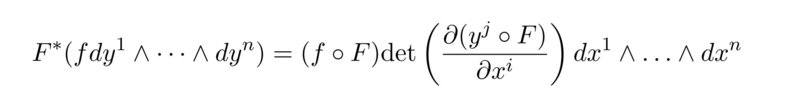# Pullback on a manifold

• A
Hey,
we had in the lecures the following:
Let M and N be smooth manifolds, and dim(M)=dim(N)=n, while $$x^i$$ and $$y^i$$ are coordinate functions around $$p\in M$$ respective $$F(p) \in N$$, then we get for the pullback of FWhich entries has the matrix we take the determinant of? I thaught of partial derivatives but am not sure.

#### Attachments

Orodruin
Staff Emeritus
Ok, thank you for your answer. But answer me please two last questions that arose. I deduce that these partial derivatives are defined, but they are vague in the sense, that $$x^i , y^i$$ are both functions the derivatives depend on. Is it the interest to leave them this vague or does one insert certain values so that the coordinate functions give „real" coordinates.